• torch.stcak()函数对多个张量在维度上进行叠加。 其中参数dim代表不同的维度。 具体如下代码所示： >>> a = torch.ones(3,3) >>> a tensor([[1., 1., 1.], [1., 1., 1.], [1., 1., 1.]]) >>> b = torch.ones(3,3) ...
• 一般torch.cat()是为了把函数torch.stack()得到tensor进行拼接而存在的。 区别参考链接torch.stack()，但是本文主要说cat()。 前言 和python中的内置函数cat()， 在使用和目的上，是没有区别的。 1. cat()官方解释 ...
• PyTorch学习笔记：torch.cattorch.stack——张量的拼接torch.cat()torch.stack()cat与stack的区别 torch.cat() torch.cat(tensors, dim=0, *, out=None) → Tensor 官方解释：利用给定的维度连接给定的张量序列...


PyTorch学习笔记：torch.cat与torch.stack——数组的拼接
torch.cat()torch.stack()cat与stack的区别

torch.cat()
torch.cat(tensors, dim=0, *, out=None) → Tensor

官方解释：利用给定的维度连接给定的数组序列(cat代表concatenate)，所有数组必须具有相同的形状（连接维度除外）或为空。 相当于按指定维度将数组进行拼接
参数解释：
tensors：要连接的数组序列(元组tuple或者列表list)dim：数组连接的维度out：输出数组(一般用不到，如果有输出，则可以直接进行赋值操作)
注意： ①tensors输入的必须是数组序列，不能是单个数组； ②输入的数组序列除了dim维度，其他维度必须形状相同。
举例：
import torch
a=torch.arange(6).reshape(2,3)
b=torch.arange(12)
c=torch.cat((a,b.reshape(4,3)),dim=0)
# 沿第0维度进行拼接，也就是按行拼接(竖着拼)
d=torch.cat((a,b.reshape(2,6)),dim=1)
# 沿第1维度进行拼接，也就是按列拼接(横着拼)
print(c)
print(c.shape)
print(d)
print(d.shape)

输出：
tensor([[ 0,  1,  2],
[ 3,  4,  5],
[ 0,  1,  2],
[ 3,  4,  5],
[ 6,  7,  8],
[ 9, 10, 11]])
torch.Size([6, 3])
tensor([[ 0,  1,  2,  0,  1,  2,  3,  4,  5],
[ 3,  4,  5,  6,  7,  8,  9, 10, 11]])
torch.Size([2, 9])

利用torch.cat()沿dim拼接，在形状上看相当于对dim进行相加，其余维度大小不变，利用这个思想，可以很容易理解高维数组的拼接
高维举例：
import torch
a=torch.ones(4*256*56*56).reshape(4,256,56,56)
b=torch.arange(4*128*56*56).reshape(4,128,56,56)
c=torch.zeros(4*64*56*56).reshape(4,64,56,56)
d=torch.cat((a,b,c),dim=1)
print(d.shape)

输出：
torch.Size([4, 448, 56, 56])

上述例子在卷积神经网络中常用于特征图的堆叠。
torch.stack()
torch.stack(tensors, dim=0, *, out=None) → Tensor

官方解释：沿着新的维度连接一系列数组，所有的数组都需要具有相同的大小。 相当于先将多个n维数组进行扩维操作，然后再拼接为一个n+1维的数组
参数解释：
tensors：要连接的数组序列(元组tuple或者列表list)dim：要插入的维度，大小必须介于0和需要拼接的数组维数之间(dim最大不超过数组的维数)out：输出数组(与cat()类似，一般用不到)
注意： ①与cat类似，必须输入数组序列，不能是单个数组； ②输入的所有数组序列形状(尺寸)必须一致(这里与cat有区别)。
举例：
import torch
a=torch.arange(12).reshape(3,4)
b=torch.ones(12).reshape(3,4)
c=torch.stack((a,b),dim=0)
d=torch.stack((a,b),dim=1)
e=torch.stack((a,b),dim=2)
# dim最大可到输入数组的维数，即a、b的维数
print(c)
print(c.shape)
print(d)
print(d.shape)
print(e)
print(e.shape)

输出：
tensor([[[ 0.,  1.,  2.,  3.],
[ 4.,  5.,  6.,  7.],
[ 8.,  9., 10., 11.]],

[[ 1.,  1.,  1.,  1.],
[ 1.,  1.,  1.,  1.],
[ 1.,  1.,  1.,  1.]]])
torch.Size([2, 3, 4])
tensor([[[ 0.,  1.,  2.,  3.],
[ 1.,  1.,  1.,  1.]],

[[ 4.,  5.,  6.,  7.],
[ 1.,  1.,  1.,  1.]],

[[ 8.,  9., 10., 11.],
[ 1.,  1.,  1.,  1.]]])
torch.Size([3, 2, 4])
tensor([[[ 0.,  1.],
[ 1.,  1.],
[ 2.,  1.],
[ 3.,  1.]],

[[ 4.,  1.],
[ 5.,  1.],
[ 6.,  1.],
[ 7.,  1.]],

[[ 8.,  1.],
[ 9.,  1.],
[10.,  1.],
[11.,  1.]]])
torch.Size([3, 4, 2])

仔细观察上个案例维度的变化，可以发现当输入为两组数组时，dim定为几，拼接后哪个维度就是2(有两个输入数组)，相当于做了一个扩维拼接操作。首先按dim增加一个维度，然后再从该维度上进行拼接操作。
cat与stack的区别
torch.cat()是直接在原数组数据上进行拼接，不会改变维数大小；torch.stack首先进行扩维，然后再进行拼接，会将维数增大一个单位。
官方文档

torch.cat():https://pytorch.org/docs/stable/generated/torch.cat.html torch.stack():https://pytorch.org/docs/stable/generated/torch.stack.html

点个赞支持一下吧
展开全文pytorch python
• 1、torch.chunk 首先看官方文档的解释： 功能：尝试将张量拆分为指定数量的数据块，每个数据块都是输入张量的一个视图。 例子： >>> torch.arange(5).chunk(3) (tensor([0, 1]), tensor([2, 3]), ...
1、torch.chunk
首先看官方文档的解释：功能：尝试将张量拆分为指定数量的数据块，每个数据块都是输入张量的一个视图。
例子：
>>> torch.arange(5).chunk(3)
(tensor([0, 1]),
tensor([2, 3]),
tensor())

>>> torch.arange(6).chunk(3)
(tensor([0, 1]),
tensor([2, 3]),
tensor([4, 5]))

>>> torch.arange(7).chunk(3)
(tensor([0, 1, 2]),
tensor([3, 4, 5]),
tensor())
通过例子很好理解，torch.chunk是将tensor切割为k个tensor。
2、torch.split
首先看官方文档的解释：功能：将张量分成数据块，每个数据块都是原始张量的视图。
官方解释的功能与torch.chunk大致相同，具体的区别通过例子很容易理解。
例子：
>>> a = torch.arange(10).reshape(5,2)
>>> a
tensor([[0, 1],
[2, 3],
[4, 5],
[6, 7],
[8, 9]])

>>> torch.split(a, 2)
(tensor([[0, 1],
[2, 3]]),
tensor([[4, 5],
[6, 7]]),
tensor([[8, 9]]))

>>> torch.split(a, [1,2])
(tensor([[0, 1]]),
tensor([[2, 3],
[4, 5],
[6, 7],
[8, 9]]))
如果split_size_or_sections传入的是整数的话，就是沿着dim方向尽量分割出长度为split_size_or_sections的tensor。
如果split_size_or_sections传入的是list的话，就按照list的参数分割不同长度的tensor。
注：chunk是指定分割数量，split是指定分割完的tensor的样式。
3、torch.cat
首先看官方文档的解释：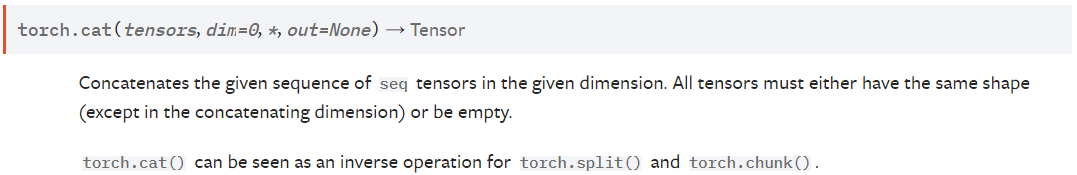功能：在给定维度上对输入的张量序列seq 进行连接操作，所有的张量必须有相同的形状（连接维度除外）或为空。
torch.cat()可以被看作是torch.split()和torch.chunk()的逆向操作。
例子：
>>> a = torch.arange(6).reshape(2, 3)
>>> a
tensor([[0, 1, 2],
[3, 4, 5]])

>>> torch.cat((a, a, a), 0)
tensor([[0, 1, 2],
[3, 4, 5],
[0, 1, 2],
[3, 4, 5],
[0, 1, 2],
[3, 4, 5]])

>>> torch.cat((a, a, a), 1)
tensor([[0, 1, 2, 0, 1, 2, 0, 1, 2],
[3, 4, 5, 3, 4, 5, 3, 4, 5]])
通过例子很好理解，torch.cat将tensor沿着dim进行拼接。
4、torch.stack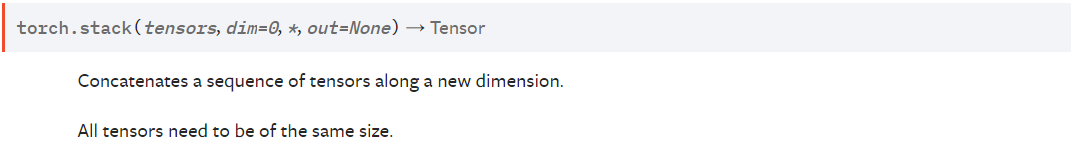功能：沿着一个新的维度连接一系列张量。所有张量需要是相同的大小。
例子：
>>> a = torch.arange(6).reshape(2, 3)
>>> a
tensor([[0, 1, 2],
[3, 4, 5],
[6, 7, 8]])

>>> torch.stack((a, a), 0) # torch.Size([2, 3, 3])
tensor([[[0, 1, 2],
[0, 1, 2]],

[[3, 4, 5],
[3, 4, 5]],

[[6, 7, 8],
[6, 7, 8]]])

>>> torch.stack((a, a), 1) # torch.Size([3, 2, 3])
tensor([[[0, 1, 2],
[0, 1, 2]],

[[3, 4, 5],
[3, 4, 5]]])

>>> torch.stack((a, a), 2) # torch.Size([3, 3, 2])
tensor([[[0, 0],
[1, 1],
[2, 2]],

[[3, 3],
[4, 4],
[5, 5]],

[[6, 6],
[7, 7],
[8, 8]]])
与cat相比不同的是，stack是在添加一个新的维度去连接，连接方式通过例子中的output和Size很容易理解。 
展开全文pytorch 深度学习 神经网络
• torch.stack，torch.cat, torch.stack.max/mean/sumtorch.stack创建两个[x,x,x,x]tensor变量torch.stack([x,x], dim=0)torch.stack([x,x], dim=1)torch.stack([x,x], dim=2)torch.stack([x,x], dim=3)torch.stack([x...


torch.stack，torch.cat, torch.stack.max/mean/sum
torch.stack创建两个[x,x,x,x]tensor变量torch.stack([x,x], dim=0)torch.stack([x,x], dim=1)torch.stack([x,x], dim=2)torch.stack([x,x], dim=3)torch.stack([x,x], dim=4)简单总结
torch.cattorch.cat([x,x], dim=0)torch.cat([x,x], dim=1)torch.cat([x,x], dim=2)torch.cat([x,x], dim=3)
torch.stack([x, x],dim=x).max(dim)torch.stack([x, x],dim=x).mean(dim)torch.stack([x, x],dim=x).sum(dim)

学习pytorch时，总免不了遇到stack和cat操作，或者有时候搞不清楚stack后max/mean/sum的操作原理。我通过代码实验为大家解说一些这些操作的原理。

torch.stack
torch.stack说白了原理也是来自于numpy.satck的操作。 主要接受两个参数： 一个数组（包含了具有相同维度的tensor元素）； 一个维度（制定了堆叠的维度位置）
一般因为我们深度学习中一般都是会用到四个维度信息，所以在这里我们也以创建具有四个维度信息的tensor变量来做说明。
创建两个[x,x,x,x]tensor变量
import torch
a = torch.FloatTensor([[[[1,2,3],[4,5,6],[7,8,9]],[[10,11,12],[13,14,15],[16,17,18]]]])
b = torch.FloatTensor([[[[19,20,21], [22,23,24],[25,26,27]],[[28,29,30],[31,32,33],[34,35,36]]]])
print(a, b, a.data.shape, b.data.shape, sep='\n')


shape信息都是[1, 2, 3, 3]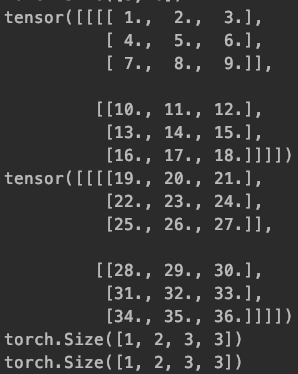torch.stack([x,x], dim=0)
对于四维信息，可以指定dim=0-4共五个维度进行堆叠。
c=torch.stack([a,b], dim=0)
print(c, c.data.shape, sep='\n')

shape信息变为[2, 1, 2, 3, 3] 在堆叠之后的tensor第一个维度将两个tensor（a和b）堆叠 其实就是相当于直接: c=[a, b], shape为[2, 1, 2, 3, 3]，如此改变了第一个维度信息，即dim=0；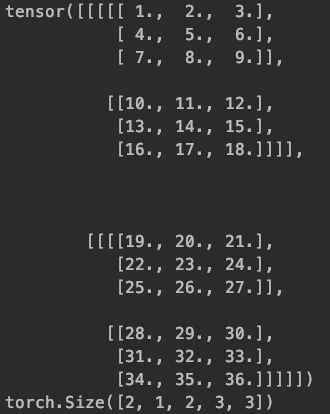torch.stack([x,x], dim=1)
c=torch.stack([a,b], dim=1)
print(c, c.data.shape, sep='\n')

shape信息变为[1, 2, 2, 3, 3] 在堆叠之后的tensor第二个维度将两个tensor（a和b）堆叠 其实就是相当于: a=（a去掉最外侧维度信息），就是变为[2,3,3] b=（b去掉最外侧维度信息），就是变为[2,3,3]
c=[a,b]， shape为[2, 2, 3, 3]，如此改变了第二个维度信息，即dim=1；
c=[c]=[[a,b]], shape为[1, 2, 2, 3, 3]，恢复dim=0时的1维度信息；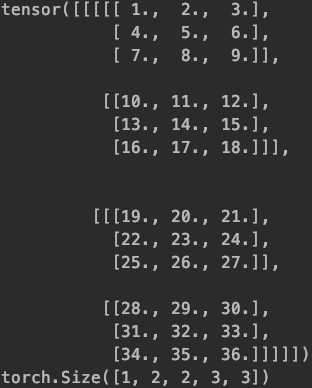torch.stack([x,x], dim=2)
c=torch.stack([a,b], dim=2)
print(c, c.data.shape, sep='\n')

shape信息变为[1, 2, 2, 3, 3] 在堆叠之后的tensor第三个维度将两个tensor（a和b）堆叠 其实就是相当于: a=（a去掉外侧维度信息），就是变为两个tensor， a1=[3,3]， a2=[3,3]； b=（b去掉外侧维度信息），就是变为两个tensor， b1=[3,3]， b2=[3,3]；
c1=[a1,b1], 如此改变了第三个维度信息，shape为[2, 3, 3]， 即dim=2 c2=[a2,b2], 如此改变了第三个维度信息，shape为[2, 3, 3]， 即dim=2
c=[c1, c2]， shape为[2, 2, 3, 3]. 恢复dim=1时的2维度信息。 c=[c]=[[c1,c2]], shape为[1, 2, 2, 3, 3].恢复dim=0时的1维度信息。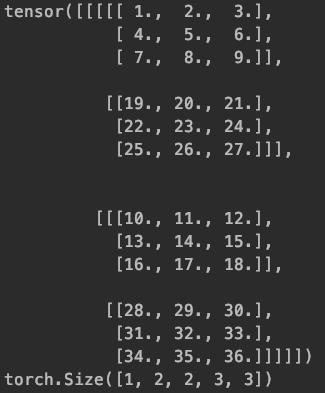torch.stack([x,x], dim=3)
c=torch.stack([a,b], dim=3)
print(c, c.data.shape, sep='\n')

shape信息变为[1, 2, 2, 3, 3] 在堆叠之后的tensor第四个维度将两个tensor（a和b）堆叠 其实就是相当于: a=（a去掉外侧维度信息），就是变为六个tensor： a1=， a2=，a3=， a4=，a5=， a6=； b=（b去掉外侧维度信息），就是变为六个tensor： b1=， b2=，b3=， b4=，b5=， b6=；
c1=[a1,b1], 如此改变了第四个维度信息，shape为[2, 3]， 即dim=3 c2=[a2,b2], 如此改变了第四个维度信息，shape为[2, 3]， 即dim=3 c3=[a3,b3], 如此改变了第四个维度信息，shape为[2, 3]， 即dim=3 c4=[a4,b4], 如此改变了第四个维度信息，shape为[2, 3]， 即dim=3 c5=[a5,b5], 如此改变了第四个维度信息，shape为[2, 3]， 即dim=3 c6=[a6,b6], 如此改变了第四个维度信息，shape为[2, 3]， 即dim=3
c1=[c1,c2,c3] ， shape 为[3, 2, 3]. 恢复dim=2时的3维度信息； c2=[c4,c5,c6]， shape 为[3, 2, 3]. 恢复dim=2时的3维度信息；
c=[c1, c2]， shape为[2, 2, 3, 3]. 恢复dim=1时的2维度信息； c=[c]=[[c1,c2]], shape为[1, 2, 2, 3, 3]. 恢复dim=0时的1维度信息；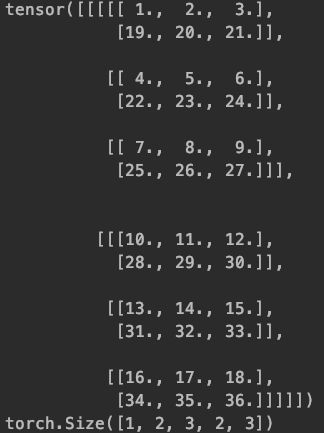torch.stack([x,x], dim=4)
c=torch.stack([a,b], dim=4)
print(c, c.data.shape, sep='\n')

shape信息变为[1, 2, 2, 3, 3] 在堆叠之后的tensor第五个维度将两个tensor（a和b）堆叠 其实就是相当于: a=（a去掉外侧维度信息），就是变为18个值： a1=1， a2=2，a3=3， a4=4，a5=5， a6=6…； b=（b去掉外侧维度信息），就是变为18个值： b1=19， b2=20，b3=21， b4=22，b5=23， b6=24…；
c1=[a1,b1], 如此改变了第 五个维度信息，shape为， 即dim=4 c2=[a2,b2], 如此改变了第五个维度信息，shape为， 即dim=4 c3=[a3,b3], 如此改变了第五个维度信息，shape为， 即dim=4 c4=[a4,b4], 如此改变了第五个维度信息，shape为， 即dim=4 c5=[a5,b5], 如此改变了第五个维度信息，shape为， 即dim=4 c6=[a6,b6], 如此改变了第五个维度信息，shape为， 即dim=4 …
c1=[c1,c2,c3] ， shape 为[3, 2]. 恢复dim=3时的3维度信息； c2=[c4,c5,c6]， shape 为[3, 2]. 恢复dim=3时的3维度信息； c3=[c7,c8,c9] ， shape 为[3, 2]. 恢复dim=3时的3维度信息； c4=[c10,c11,c12]， shape 为[3, 2]. 恢复dim=3时的3维度信息； c5=[c13,c14,c15] ， shape 为[3, 2]. 恢复dim=3时的3维度信息； c6=[c16,c17,c18]， shape 为[3, 2]. 恢复dim=3时的3维度信息； …
c1=[c1, c2, c3]，shape 为[2, 3, 2]. 恢复dim=2时的3维度信息； c2= [c4, c5, c6]，shape 为[2, 3, 2]. 恢复dim=2时的3维度信息；
c=[c1, c2 ]， shape为[2, 3, 3, 2]. 恢复dim=1时的2维度信息； c=[c]=[[c1,c2]], shape为[1, 2, 2, 3, 3]. 恢复dim=0时的1维度信息；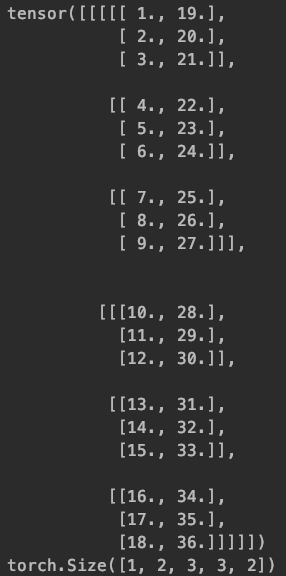简单总结
就是stack操作是将tensor先拆解到指定的维度上，再该维度上进行堆叠，然后逐渐恢复之前的维度信息。
torch.cat
对于四维信息，可以指定dim=0-3共四个维度进行concat。
c = torch.cat([a, b],dim=0)  #两个的每一列加在一起
print(c, c.data.shape, sep='\n')
c = torch.cat([a, b],dim=1)  #两个的每一列加在一起
print(c, c.data.shape, sep='\n')
c = torch.cat([a, b],dim=2)  #两个的每一列加在一起
print(c, c.data.shape, sep='\n')
c = torch.cat([a, b],dim=3)  #两个的每一列加在一起
print(c, c.data.shape, sep='\n')

原理和stack感觉差不多，只不过stack是堆叠出一个新的维度信息，由4个维度变为五个维度，而cat是四个维度依然是四个维度，只不过制定维度位置上的channels直接进行了翻倍或者多倍。

torch.cat([x,x], dim=0)
shape信息变为[2, 2, 3, 3] 其实就是相当于直接: a=[2,3,3] b=[2,3,3] c=[a, b], shape为[2, 2, 3, 3]，如此翻倍了第一个维度信息，即dim=0；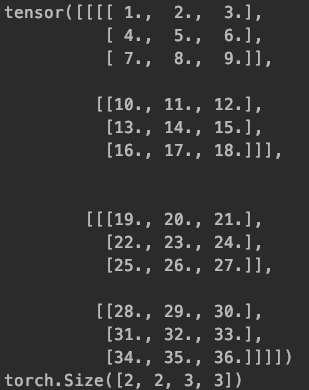torch.cat([x,x], dim=1)
shape信息变为[1, 4, 3, 3] 其实就是相当于直接: a1=[3,3]，a2=[3,3] b1=[3,3]，b2=[3,3]， c=[a1,a2,a3,a4], shape为[4, 3, 3]，如此翻倍了第二个维度信息，即dim=1； c=[c], 恢复第一个维度信息1，shape为[1, 4, 3, 3]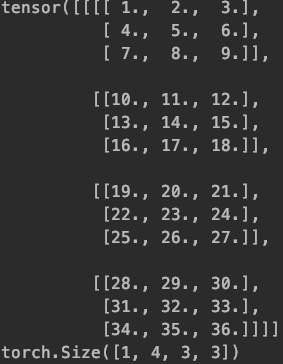torch.cat([x,x], dim=2)
shape信息变为[1, 2, 6, 3] 其实就是相当于直接: a=（a去掉外侧维度信息），就是变为六个tensor： a1=， a2=，a3=， a4=，a5=， a6=； b=（b去掉外侧维度信息），就是变为六个tensor： b1=， b2=，b3=， b4=，b5=， b6=； c1=[a1,a2,a3,b1,b2,b3], shape为[6, 3]，如此翻倍了第三个维度信息，即dim=2； c2=[a4,a5,a6,b4,b5,b6], shape为[6, 3]，如此翻倍了第三个维度信息，即dim=2； c=[c1,c2], 恢复第二个维度信息2，shape为[2, 6, 3] c=[c], 恢复第一个维度信息1，shape为[1, 2, 6, 3]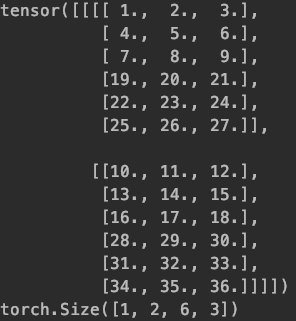torch.cat([x,x], dim=3)
a=（a去掉外侧维度信息），就是变为18个值： a1=1， a2=2，a3=3， a4=4，a5=5， a6=6…； b=（b去掉外侧维度信息），就是变为18个值： b1=19， b2=20，b3=21， b4=22，b5=23， b6=24…；
然后先将a1,a2,a3,b1,b2,b3放入一个[]，翻倍了维度， 如此进行，一共将6个[]进行了翻倍，shape为 再将前三个放入[], 恢复第3个维度信息, shape为[3, 6] 再将得到的两个[]放入[], 恢复第2个维度信息, shape为[2, 3, 6] 再将得到的一个[]放入[], 恢复第1个维度信息, shape为[1, 2, 3, 6]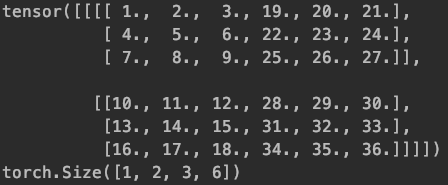torch.stack([x, x],dim=x).max(dim)
max中的dim也可以指定dim。 我们以torch.stack([a, b],dim=1).max(dim) 来解说一下操作。 同样对于max，其中的维度可以指定五个（对于四个维度信息来说）
c,_=torch.stack([a,b], dim=1).max(0)
print('dim=0 ', c, c.data.shape, sep='\n')
c,_=torch.stack([a,b], dim=1).max(1)
print('dim=1 ', c, c.data.shape, sep='\n')
c,_=torch.stack([a,b], dim=1).max(2)
print('dim=2 ', c, c.data.shape, sep='\n')
c,_=torch.stack([a,b], dim=1).max(3)
print('dim=3 ', c, c.data.shape, sep='\n')
c,_=torch.stack([a,b], dim=1).max(4)
print('dim=4 ', c, c.data.shape, sep='\n')

其实就是在相应的维度上找最大值，然后维度信息会恢复到堆叠前的维度信息。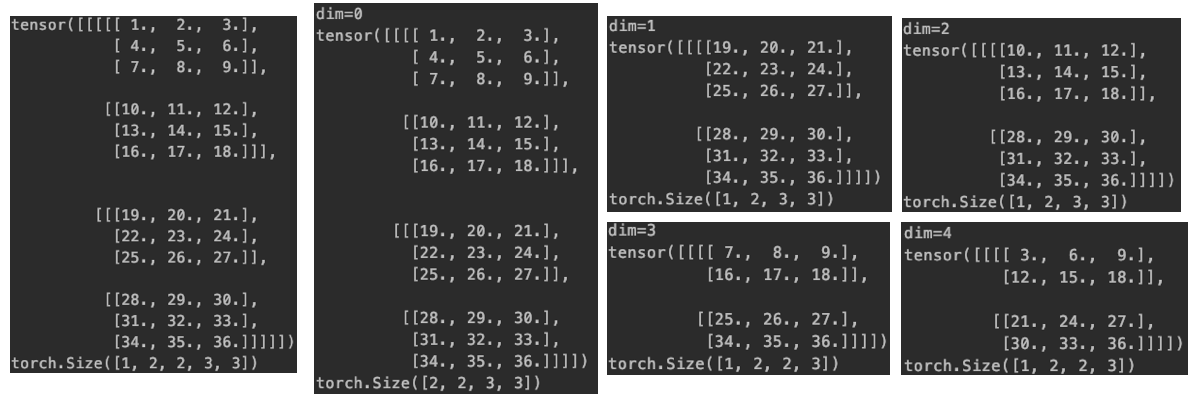torch.stack([x, x],dim=x).mean(dim)
mean中的dim也可以指定dim。 我们以torch.stack([a, b],dim=1).mean(dim=1) 来解说一下操作。 同样对于mean，其中的维度可以指定五个（对于四个维度信息来说）
c=torch.stack([a,b], dim=1).mean(1)
print('dim=1 ', c, c.data.shape, sep='\n')

其实就是在相应的维度上进行element-wise加和，再取average，然后维度信息会恢复到堆叠前的维度信息。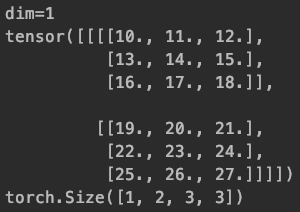torch.stack([x, x],dim=x).sum(dim)
sum中的dim也可以指定dim。 我们以torch.stack([a, b],dim=1).sum(dim=1) 来解说一下操作。 同样对于sum，其中的维度可以指定五个（对于四个维度信息来说）
c=torch.stack([a,b], dim=1).sum(1)
print('dim=1 ', c, c.data.shape, sep='\n')

其实就是在相应的维度上进行element-wise加和，不再取average，然后维度信息会恢复到堆叠前的维度信息。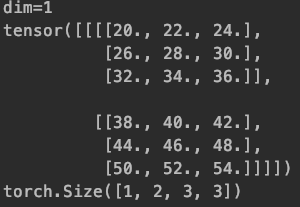2020.03.25

展开全文python 深度学习 numpy 神经网络
• torch.stack()和torch.cat()都是拼接tensor常用操作，stack()可以看做并联，cat()为串联。 torch.stack() 官网：https://pytorch.org/docs/stable/torch.html#torch.stack torch.stack(tensors,dim=0,out=None)...
torch.stack() 和 torch.cat() 都是拼接tensor常用操作，stack()可以看做并联，cat()为串联。
torch.stack()
官网：https://pytorch.org/docs/stable/torch.html#torch.stack

torch.stack(tensors, dim=0, out=None) → Tensor

torch.stack() 将序列连接，形成一个新的tensor结构，此结构中会增加一个维度。连接中的 每个tensor都要保持相同的大小。
参数：
tensors：需要连接的结构 ；dim：需要扩充的维度 ；output：输出的结构
例子：
import torch

l = []
for i in range(0,3):
x = torch.rand(2,3)
l.append(x)
print(l)

x = torch.stack(l,dim=0)

print(x.size())

z = torch.stack(l,dim=1)
print(z.size())

output：
[tensor([[0.3615, 0.9595, 0.5895],
[0.8202, 0.6924, 0.4683]]), tensor([[0.0988, 0.3804, 0.5348],
[0.0712, 0.4715, 0.1307]]), tensor([[0.1635, 0.4716, 0.1728],
[0.8023, 0.9664, 0.4934]])]
torch.Size([3, 2, 3])
torch.Size([2, 3, 3])
torch.cat()
官网：https://pytorch.org/docs/stable/torch.html#torch.cat

torch.cat(tensors, dim=0, out=None) → Tensor

参数：同上，tensor必须是相同的维度。
>>> x = torch.randn(2, 3)
>>> x
tensor([[ 0.6580, -1.0969, -0.4614],
[-0.1034, -0.5790,  0.1497]])
>>> torch.cat((x, x, x), 0)
tensor([[ 0.6580, -1.0969, -0.4614],
[-0.1034, -0.5790,  0.1497],
[ 0.6580, -1.0969, -0.4614],
[-0.1034, -0.5790,  0.1497],
[ 0.6580, -1.0969, -0.4614],
[-0.1034, -0.5790,  0.1497]])
>>> torch.cat((x, x, x), 1)
tensor([[ 0.6580, -1.0969, -0.4614,  0.6580, -1.0969, -0.4614,  0.6580,
-1.0969, -0.4614],
[-0.1034, -0.5790,  0.1497, -0.1034, -0.5790,  0.1497, -0.1034,
-0.5790,  0.1497]])
>>> torch.cat(((x,x),x),1) ->报错,不同维度
区别：
下面例子说明torch.cat()与torch.stack()区别。可以看出，stack()是增加新的维度来完成拼接，不改变原维度上的数据大小。cat()是在现有维度上进行数据的增加（改变了现有维度大小），不增加新的维度。
x = torch.rand(2,3)
y = torch.rand(2,3)
print(torch.stack((x,y),1).size())
print(torch.cat((x,y),1).size())

output：
torch.Size([2, 2, 3])
torch.Size([2, 6])


展开全文• torch.cat(tensors, dim=0, out=None) → Tensor Concatenates the given sequence of seq tensors in the given dimension. All tensors must either have the same shape (except in the concatenating ...
• 网上很多的示例，都在讨论二...torch.cat这是一个拼接函数（姑且这么说） 直接上例子 a0=torch.Tensor([[[[1,1,1,1],[2,2,2,2]]]]) a1=torch.Tensor([[[[3,3,3,3],[4,4,4,4]]]]) torch.Size([1, 1, 2, 4]) torch.ca
• torch.cattorch.stack看起来相似但是性质还是不同的 使用python中的list列表收录tensor时，然后将list列表转化成tensor时，会报错。这个时候就要使用torch.stack进行堆叠，转化成tensor。 torch.cat() torch....
• torch.cat(dim=0) = torch.vstack() torch.cat(dim=1) = torch.hstack() 除了torch.stack(),dim=1,dim=2有点怪之外，其他的都是正常操作
• 维度转换 在torch中应用卷积的时候，我们有...y0 = torch.cat((x0, x1),0).size() print(y0) y1 = torch.add(x0,x1).size() print(y1) 输出结果 2 维度变换 尺度对应，当然tensor.view()还有其他用法，具体参考源码 //python pytorch
• 部分内容摘自pytorch中文文档 torch.cat torch.cat(inputs, ...torch.cat()可以看做 torch.split() 和 torch.chunk()的反操作， cat() 函数可以通过下面例子更好的理解。 参数： inputs (sequence of Tenso...
• 目录1 torch.cat()2 torch.split() 1 torch.cat() torch.cat(tensors, dim=0, *, out=None) → Tensor Concatenates the given sequence of seq tensors in the given dimension. All tensors must either have ...pytorch python 深度学习
• 1、关于torch.stack做拼接的时候，无论是按照那个维度进行拼接，其结果都会进行扩维的，下面来看具体的例子： import torch a=torch.tensor([[1,2,3],[4,5,6]]) b=torch.tensor([[7,8,9],[10,11,12]]) c=torch.stack...深度学习 神经网络 人工智能
• 一、torch.cat()函数 熟悉C字符串的同学们应该都用过strcat（）函数，这个函数在C/C++程序中用于连接2个C字符串。在pytorch中，同样有这样的函数，那就是torch.cat()函数. 先上源码定义：torch.cat(tensors,dim=0,...计算机视觉 深度学习 机器学习
• 用法： torch.stack((tensor1,tensor2),dim=?...torch.stack和torch.cat都是用于拼接的，核心不同在于使用stack后，原来的张量会增加一维，比如把两个3 * 3（二维）的tensor用torch.stack在dim0拼接，pytorch 经验分享 机器学习
• torch.stack torch.stack(inputs, ...torch.cat torch.cat(inputs, dim=0) inputs：必须是张量序列， 在给定维度上对输入的张量序列进行连接操作，序列中所有的张量都应该为相同形状。 #注:由字面意思可以看出， c...深度学习 机器学习
• Pytorch阅读文档之torch.cat (),torch.split() and torch.chunk()函数 torch.cat ()函数 torch.cat(tensors, dim=0, out=None) → Tensor #在给定维度上连接给定序列张量的序列。 #所有张量必须具有相同的形状（在...Pytorch
• torch.cat() 和 torch.stack()略有不同 torch.cat(tensors,dim=0,out=None)→ Tensor torch.cat()对tensors沿指定维度拼接，但返回的Tensor的维数不会变 torch.stack(tensors,dim=0,out=None)→ Tensor torch.stack...python
• torch.cat是将两个张量（tensor）拼接在一起，cat是concatnate的意思，即拼接，联系在一起。 2. 例子理解 >>> import torch >>> A=torch.ones(2,3) #2x3的张量（矩阵） >>> A tensor([[ 1., 1., 1.], [ 1., 1., 1....
• 【pytorch】torch.cattorch.stack的区别 torch.cat import torch a=torch.randn((1,3,4,4)) #假设代表了[N,c,w,h] b=torch.cat((a,a)) #维度默认是0 # (2, 3, 4, 4) c=torch.cat((a,a),dim=1) # (1, 6, 4, 4) ...
• 1、torch.rand(*sizes, out=None)→ Tensor 均匀分布。返回一个张量，包含了从区间[0, 1)的均匀分布中抽取的一组随机数。张量的形状由参数sizes定义。 参数: sizes (int…) - 整数序列，定义了输出张量的形状 ...深度学习 pytorch 机器学习
• torch.cat 沿着特定维数连接一系列张量。 torch.stack 沿新维度连接一系列张量。 torch.cat 在给定维度中连接给定的 seq 个张量序列。 所有张量必须具有相同的形状（连接维度除外）或为空。 torch.cat(tensors, dim...pytorch 深度学习 python
• torch.cat：想要去对那哪一个维度进行concat就必须要保证其他维度的大小是一样的。 如a的shape为（2，2，3），b的shape为（3，2，3），那么在维度0上是可以进行concat的，concat过后得到（5，2，3）而在维度1和2维度...python pytorch
• 在进行cv相关实验中我们用的比较多的都是torch.cat()和torch.stack()函数。其中cat()函数的功能是在当前维度进行数据的拼接。stack()函数首先将当前维度及其以后维度的数据向后移动一位，将该位置的大小修改为1然后...pytorch
• 1torch.cat() 在给定维度上对输入的张量序列seq进行连接操作。torch.cat()可以看做 torch.split() 和 torch.chunk()的反操作。 cat() 函数可以通过下面例子更好的理解。 torch.cat(seq, dim=0, out=None) → ...
• 使用torch.cat()连接张量时，出现错误如下： TypeError: cat() received an invalid combination of arguments - got (Tensor, Tensor, dim=int), but expected one of: (tuple of Tensors tensors, name dim, *, ...debug pytorch
• torch.cat()函数可以将多个张量拼接成一个张量。torch.cat()有两个参数，第一个是要拼接的张量的列表或是元组；第二个参数是拼接的维度。 torch.cat()的示例如下图1所示 图1 torch.cat() torch.stack()函数...Pytorch Tensor
• import torch a = torch.randn((2, 3, 4)) print(a) res: tensor([[[ 0.2615, 0.9965, 0.5935, -2.4657], [-2.0211, 0.5055, 0.3128, -0.8843], [-1.3269, -1.0438, 0.3159, -0.8572]], [[ 0.7990, 0.2023, -0......